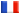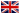# Séminaire de Cryptographie

## Joost Renes### Complete Addition Formulas for Prime Order Elliptic Curves

An elliptic curve addition law is said to be complete if it correctly computes the sum of any two points in the elliptic curve group. One of the main reasons for the increased popularity of Edwards curves in the ECC community is that they can allow a complete group law that is also relatively efficient (e.g., when compared to all known addition laws on Edwards curves). Such complete addition formulas can simplify the task of an ECC implementer and, at the same time, can greatly reduce the potential vulnerabilities of a cryptosystem. Unfortunately, until now, complete addition laws that are relatively efficient have only been proposed on curves of composite order and have thus been incompatible with all of the currently standardized prime order curves.

In this paper we present optimized addition formulas that are complete on every prime order short Weierstrass curve defined over a field k with char(k) not 2 or 3. Compared to their incomplete counterparts, these formulas require a larger number of field additions, but interestingly require fewer field multiplications. We discuss how these formulas can be used to achieve secure, exception-free implementations on all of the prime order curves in the NIST (and many other) standards.